虚数的意义

"我一直觉得虚数（imaginary number）很难懂。

中学老师说，虚数就是-1的平方根。可是，什么数的平方等于-1呢？计算器直接显示出错！

直到今天，我也没有搞懂。谁能解释，虚数到底是什么？

它有什么用？"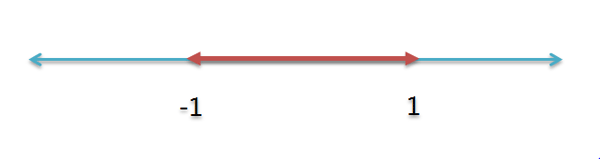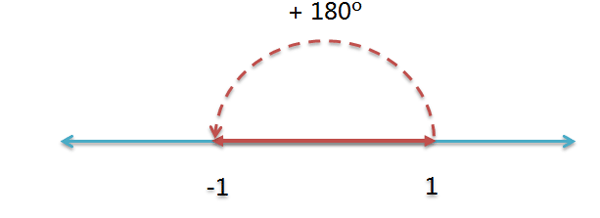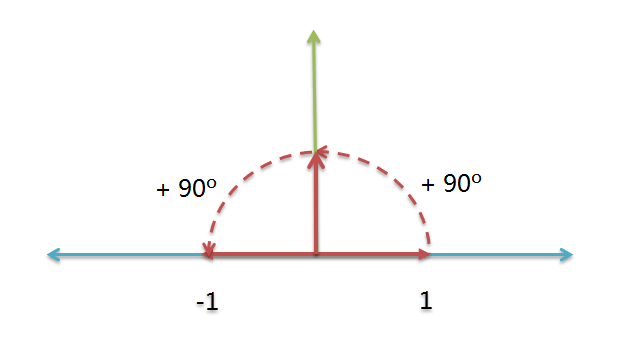(+1) * (逆时针旋转90度) * (逆时针旋转90度) = (-1)

(逆时针旋转90度)^2 = (-1)

i^2 = (-1)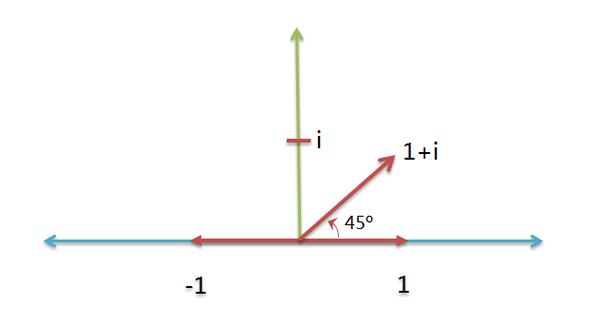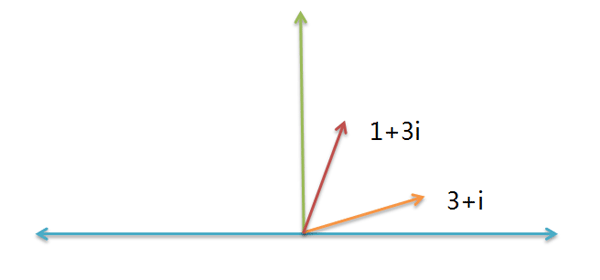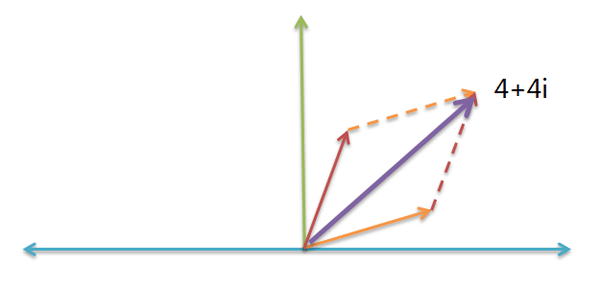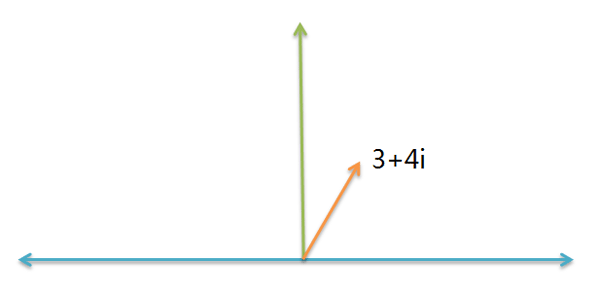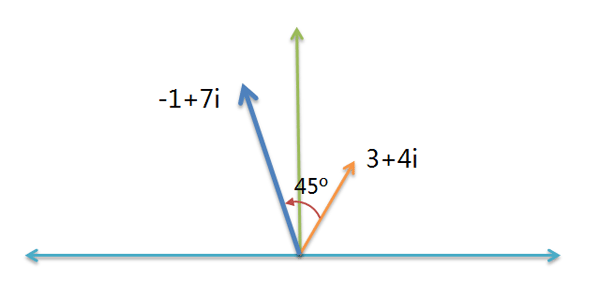45度的航向就是 1 + i 。计算新航向，只要把这两个航向 3 + 4i 与 1 + i 相乘就可以了（原因在下一节解释）：

( 3 + 4i ) * ( 1 + i ) = ( -1 + 7i )

( 3 + 4i ) * i = ( -4 + 3i )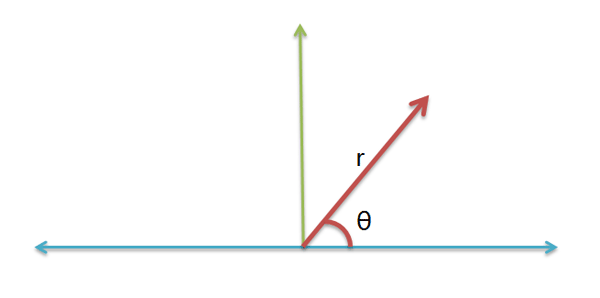a + bi = r1 * ( cosα + isinα )

c + di = r2 * ( cosβ + isinβ )

r1 * r2 * ( cosα + isinα ) * ( cosβ + isinβ )

cosα * cosβ - sinα * sinβ + i( cosα * sinβ + sinα * cosβ )

cos(α+β) + isin(α+β)

( a + bi )( c + di )　＝　r1 * r2 * ( cos(α+β) + isin(α+β) )